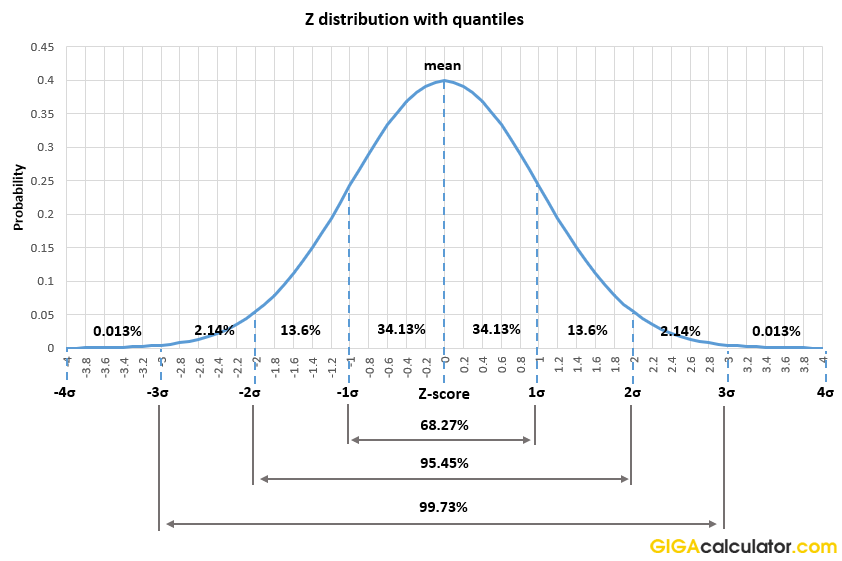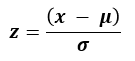# Z Score Calculator

Use this Z table calculator to easily calculate the Z-score from a given raw score. Also computes areas under the normal curve (p-values) cut off by a given score. A table of Z scores and corresponding p-values is included, as well as the z score formula. Also calculates Z from p.

x=
μ=
σ=
Share calculator:

Embed this tool:
get code

## Using the Z score calculator

The z score calculator can be used to derive a z statistic from a raw score and known or estimated distribution mean and standard deviation. If the variance is known instead, then the standard deviation is simply its square root. The output also contains probabilities calculated for different areas under the standard normal curve which correspond to a one-tailed or two-tailed test of significance. The cumulative probabilities are calculated using the standard normal cumulative distribution function (CDF).

The z statistic calculator can also be used in inverse - to obtain a Z critical value corresponding to a given probability. Simply select "Z score from P" and enter the p-value threshold in the field to obtain the standard score defining the critical region.

## What is a Z Distribution?

The Z distribution is simply the standard normal distribution of the random variable Z meaning it is a normal distribution with mean 0 and variance and standard deviation equal to 1 [1,2,3]. The Z distribution with key quantiles is shown on the graph below:The fact that the distribution is standardized means that the quantiles are known, and that area between any two Z scores is also known. For example, 68.27% of values would fall between -1 and 1 standard deviations of a Z distribution. Similarly, just over 95% of its probability density falls between -2 and +2 standard deviations. The entire distribution density sums to 1 and just like other normal distributions it is fully defined by its first two moments. These and other qualities make it a useful tool in statistics and probability calculation of various sorts.

Our z score calculator uses the CDF of the Z distribution to find the area under the standard normal curve above, below, between, or outside regions defined by given scores. It uses the inverse CDF to calculate Z scores from p-values.

## What is a "Z score"?

The z-score, also referred to as standard score and z-value is a signed real valued dimensionless quantity which indicates the number of standard deviations by which a given observed data point is distanced from the mean or expected value of a distribution. Standard refers to the fact that they are computed against the standard normal distribution (a.k.a. Z distribution) which is fully defined by its mean and standard deviation of zero and one, respectively.

Z values have numerous applications in statistical inference and estimation. Most commonly they are used in a Z-test of significance as well as confidence interval calculations . They are also used in process control and quality assurance applications (e.g. six sigma). Due to Z values being standardized scores they are useful in comparing measurements across different scales which is often needed in both scientific and applied disciplines.

## Z score formula

The formula for calculating a z score from a raw score from is given by the simple equation:Using the above formula one can easily convert a raw score from a normal distribution with known or estimated mean and standard deviation to a standard score. An online Z calculator can perform the arithmetic for you quickly and easily.

## Z table

A Z table contains tabulated values of the Z distribution and their corresponding quantiles, or percentages. Since a table of Z scores can be used to judge either a point null hypothesis (e.g. the effect is exactly zero), as well as the more commonly needed composite nulls such as μ1 ≤ 0, a proper Z table should contain both types of cumulative probabilities. In the table below, a one-tailed p-value / percentile refers to the area under the standard normal curve to the right of the Z score ( P(X > z) ), whereas a two-tailed p-value / percentile refers to the cumulative probability contained in the union of the areas to the right of -Z and to the left of Z ( P(X < -|z| ∪ X > |z|) ).

Table of commonly used Z-score cut-offs for defining critical regions for normally distributed random variables:

Common Z score cut-offs
Z scoreP-value (1-tailed)Percentile (1-tailed)P-value (2-tailed)Percentile (2-tailed)
0.3186 0.5000 50.00% 0.7500 25.00%
0.5000 0.3085 69.15% 0.6171 38.29%
0.6745 0.2500 75.00% 0.5000 50.00%
0.8416 0.2000 80.00% 0.4000 60.00%
1.0000 0.1587 84.13% 0.3173 68.27%
1.2816 0.1000 90.00% 0.2000 80.00%
1.6448 0.0500 95.00% 0.1000 90.00%
1.9599 0.0250 97.50% 0.0500 95.00%
2.0000 0.0228 97.72% 0.0455 95.45%
2.3263 0.0100 99.00% 0.0200 98.00%
2.5758 0.0050 99.50% 0.0100 99.00%
3.0000 0.0013 99.87% 0.0027 99.73%
3.7190 0.0010 99.99% 0.0020 99.98%
3.8900 0.000050 99.995% 0.000100 99.990%

Note that since the standard normal distribution is symmetrical, a two-tailed p-value is exactly twice that of a one-tailed one for the same value of Z. Obviously, such a table has limited utility nowadays when it is much easier to use a free online z table calculator like ours. Refer to the documentation and graphs in our critical value calculator page for more on critical values and regions.

## Example calculations

It is easy to find the Z score corresponding to a given raw score, given that one knows the mean and standard deviation of the normal distribution to which the raw score belongs. Using the z statistic formula above we can easily compute that a raw score from a standard normal distribution is equivalent to the Z score since z = (x - μ) / σ = x for μ = 0 and σ = 1.

In another example, a raw score of 1600 from a distribution with mean 1000 and variance 90,000 is given. How do we find the Z score? First, convert the variance (σ2) to standard deviation (σ) by taking its square root: σ = √90000 = 300. Then the solution is simply:

z = (1600 - 1000) / 300 = 600 / 300 = 2

This tells us that the raw score of 1600 is 2 standard deviations away from the mean. The normal CDF can then be used to arrive at whatever area under the standard normal curve is of interest. For example, to reject the hypothesis that the true value related to the observation is not lower than or equal to zero, one needs to compute

p = P(X > z) = P(X > 2) = 0.0228

which is the 2.28% percentile. For the last part one can look up the Z table for the row where Z = 2, or use a Z score probability calculator if the value is not tabulated or a greater precision is needed.